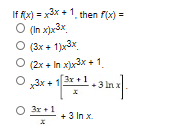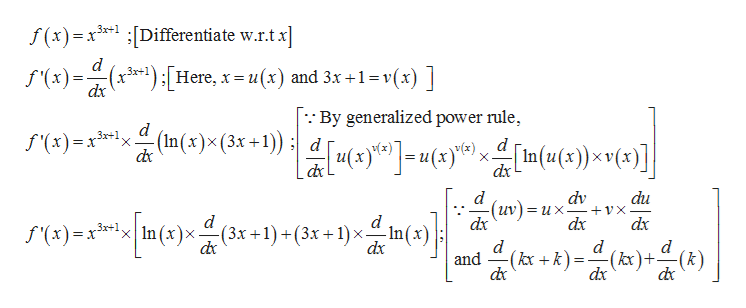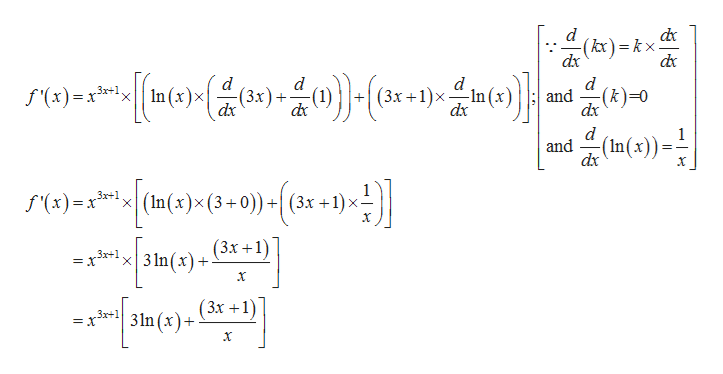# If fx)x3x+1 then fx)(In x]3x(3x+1}x3x(2x+ In xr3x+ 13x13r 3 Inx3x+13 In x

Question
3 viewshelp_outlineImage TranscriptioncloseIf fx)x3x+1 then fx) (In x]3x (3x+1}x3x (2x+ In xr3x+ 1 3x13r 3 Inx 3x+13 In x fullscreen
check_circle

Step 1

Note:

Hi there! Thank you for posting the question. The first line in the image is not very clear. However, on comparing it with the below given options, we have found it logical to assume that the question enquires about f’(x). We are finding the derivative of the given function f(x).

Step 2

Obtain the derivative f’(x):

The given function is f(x) = x3x+1.

The derivative f’(x) is obtained as given below:help_outlineImage Transcriptionclosef(x)xDifferentiate w.r.tx 3x+ d (x3-1);[Here, x=u(x) and 3x +1 vx) dx f'(x) _ By generalized power rule (In(s)x (3x +1)) ;| du(x) (x) 3x+1 = X f'(x)x31, In(u()v() X d X dx d dv du (uv) dx = ux +vX dx dx f'(x)x n(x)x 3x+1 In(x) dx (3x +1)+(3x +1) x. d (kx +k (kx)+-(k) dx and fullscreen
Step 3

Further calculation:

The derivative f’(x) is obtained as x3x+1[((3x+1)/x)+3ln(x)] from the cal...help_outlineImage Transcriptionclose(kx)=kx dx dx d d d In(x)x 3x+1 = X -In (x) (3x +1)x dx (3x) (k)=0 f'(x) and dx dx d (In(x))= and dx 3x+1 (3 f'(x) (3x +1) 3 In(x) 3x+1 X X = (3x +1) 3r+1 = X 31n(x) + fullscreen

### Want to see the full answer?

See Solution

#### Want to see this answer and more?

Solutions are written by subject experts who are available 24/7. Questions are typically answered within 1 hour.*

See Solution
*Response times may vary by subject and question.
Tagged in

### Derivative